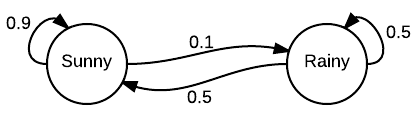# Markov Chain

## What is a Markov Chain?

Markov chains are used to model probabilities using information that can be encoded in the current state. Something transitions from one state to another semi-randomly, or stochastically.  Each state has a certain probability of transitioning to each other state, so each time you are in a state and want to transition, a markov chain can predict outcomes based on pre-existing probability data. More technically, information is put into a matrix and a vector - also called a column matrix - and with many iterations, a collection of probability vectors makes up Markov chains.  To determine the transition probabilities, you have to "train" your Markov Chain on some input corpus.

### Markov Matrix

A Markov Matrix, or stochastic matrix, is a square matrix in which the elements of each row sum to 1. It can be seen as an alternative representation of the transition probabilities of a Markov chain.

Representing a Markov chain as a matrix allows for calculations to be performed in a convenient manner. For example, for a given Markov chain P, the probability of transition from state i to state j in k steps is given by the (i, j)th element of Pk.

### Markov Model

A Markov model is a stochastic model with the property that future states are determined only by the current state -- in other words, the model has no memory; it only knows what state it's in now, not any of the states which occurred previously.

A Markov chain is one example of a Markov model, but other examples exist. One other example commonly used in the field of artificial intelligence is the Hidden Markov model, which is a Markov chain for which the state is not directly observable. Observations can be made, but these observations are not usually sufficient to uniquely determine the state of the model. Hidden Markov models are used, for example, in speech recognition: the audio waveform of the speech is the direct observation, and the actual state of the system is the spoken text.

### An Easy Example of a Markov ChainThe easiest way to explain a Markov chain is by simply looking at one. In this example, we can see we have two states: “sunny” and “rainy”. Let’s say the day is sunny, and we want to know what the chances are that it will be sunny the next day. We can see that the Markov chain indicates that there is a .9, or 90%, chance it will be sunny. Following this pattern, we can see that there will probably be many sunny days lumped together followed by a shorter string of rainy days. It is not certain, but likely.

Obviously, this is not a real world example - this is not how real-world weather models work. However, if one were building a video game that had a “real” weather component, a Markov chain like this may be useful when fed several years of real-time data.

### Applications in Artificial Intelligence

There are quite a few applications of Markov Chains to AI -- Markov Chains are useful basically when you want to model something that's in discrete states, but you don't understand how it works.

There are a few Markov chains many of us use every day in AI, one example being predictive text programs on cell phones. You’ve probably noticed that predictive text gets “smarter” as you use your phone more often, and will sometimes suggest words that are specific to you and your world. This is because the software is slowly creating a Markov chain on your patterns, and every time you type a word, it computes the probability of the next word you’re going to type using information from what you have typed before.

Markov Chains are also used in classifiers. In 1998, a team of linguists tried to use

Markov Chains to model the phonotactic constraints of Dutch. They trained the Markov Chain on a wide variety of monosyllabic Dutch words, then asked it to determine whether a large number of other monosyllabic "words" were real Dutch words or not. It performed well, scoring over 90% accuracy.

On a more humorous note, Markov Chains are also very simple to make in some programming languages such as Python, and are sometimes used to create mashups of data for laughs, like on this Twitter account.